# Gaussian Discriminant Analysis

## Introduction

By now, you all must know that a Supervised learning algorithm is a subset of machine learning algorithms. It works on labeled datasets and predicts the outputs based on various supervised algorithms. Supervised learning is divided into two categories:

1. Discriminative Learning Algorithm
2. Generative Learning Algorithm

## What is a Discriminative Learning Algorithm?

Discriminative Learning Algorithms categorize the dataset in various classes and find the decision boundary. Logistic regressionPerceptron Algorithms come under this category.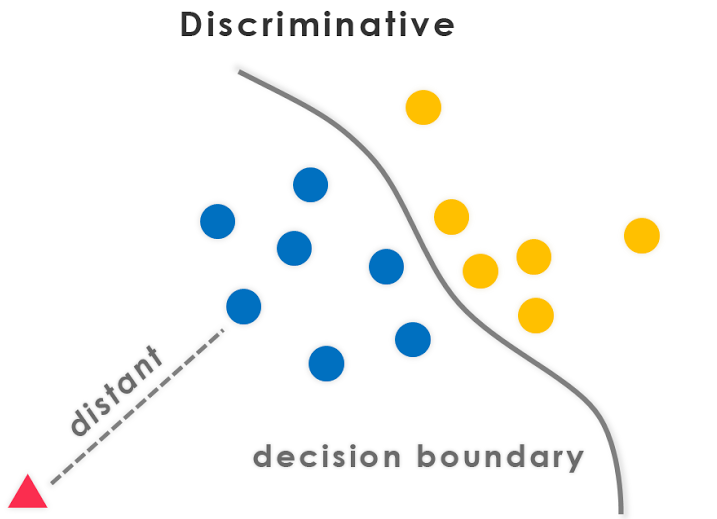## What is Generative Learning Algorithm?

Generative Learning works on a different approach; it tries to learn and build the models of all the classes separately. Naive Bayes, Linear Discriminant Analysis, Gaussian Discriminant Analysis are some examples.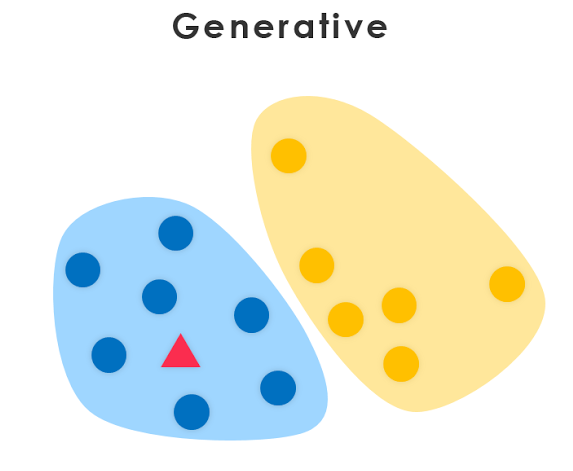In this article, we’re going to learn about Gaussian Discriminant Analysis but before that, let us first understand what discriminant analysis is.

## What is Discriminant Analysis?

Discriminant analysis is a statistical method that predicts whether data classification is sufficient or not concerning the dataset. It comes into action when

• Dependent variables are categorical.
• Independent variables are in an interval.

## Significance of Discriminant Analysis

The significance of discriminative analysis is to develop different discriminant functions. That is a linear combination of independent variables and the variables that could differentiate them from dependent variables. It can help in establishing the accuracy of the classification of two groups.

## What is Gaussian Discriminant Analysis?

Gaussian Discriminant is the subdivision of the Generative Learning Algorithm. It tries to build a model for each and every class. Although, as the name suggests, it fits the Gaussian distribution of each dataset category in the modeling process. GDA belongs to the family of classifiers, and it includes the linear and quadratic classifiers.

### Assumptions of Gaussian Discriminant Analysis

We apply Gaussian Discriminant Analysis to classify our dataset, and the input features are continuous random variables. Gaussian discriminant analysis belongs to the generative learning algorithm. We assume P(x/y) to be normally distributed and P(y) distributed according to Bernoulli’s principles.

## What is Linear Discriminant Analysis?

Linear Discriminant Analysis is a technique used to decipher two or more classes in supervised learning. It maps the linear data from a higher dimensionality to a lower dimensionality. Linear Discriminant Analysis is responsible for expressing a linear relationship between a dependent variable and other features, similar to regression analysis.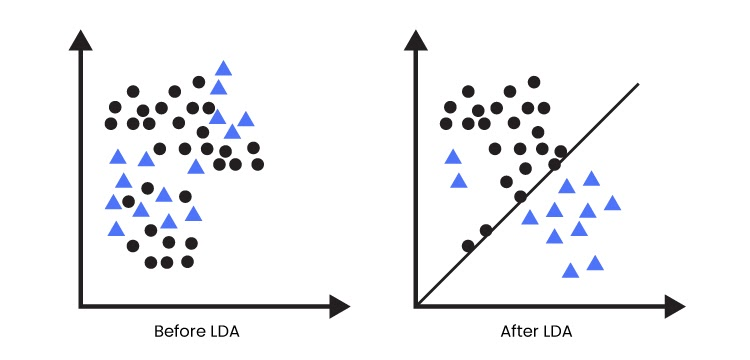## What is Quadratic Discriminant Analysis?

Quadratic Discriminant analysis presumes that every class satisfies the Gaussian distribution law. Although Quadratic Discriminant Analysis is similar to Linear Discriminant Analysis in QDA, we calculate the mean and covariance of each category separately.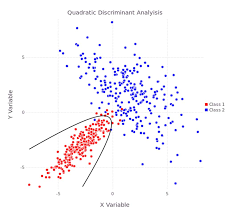## Difference between LDA and QDA

The significant difference between LDA and QDA is that we apply LDA when a linear separation occurs between the classes. In contrast, when there is a non-linear separation between the classifiers, we use QDA.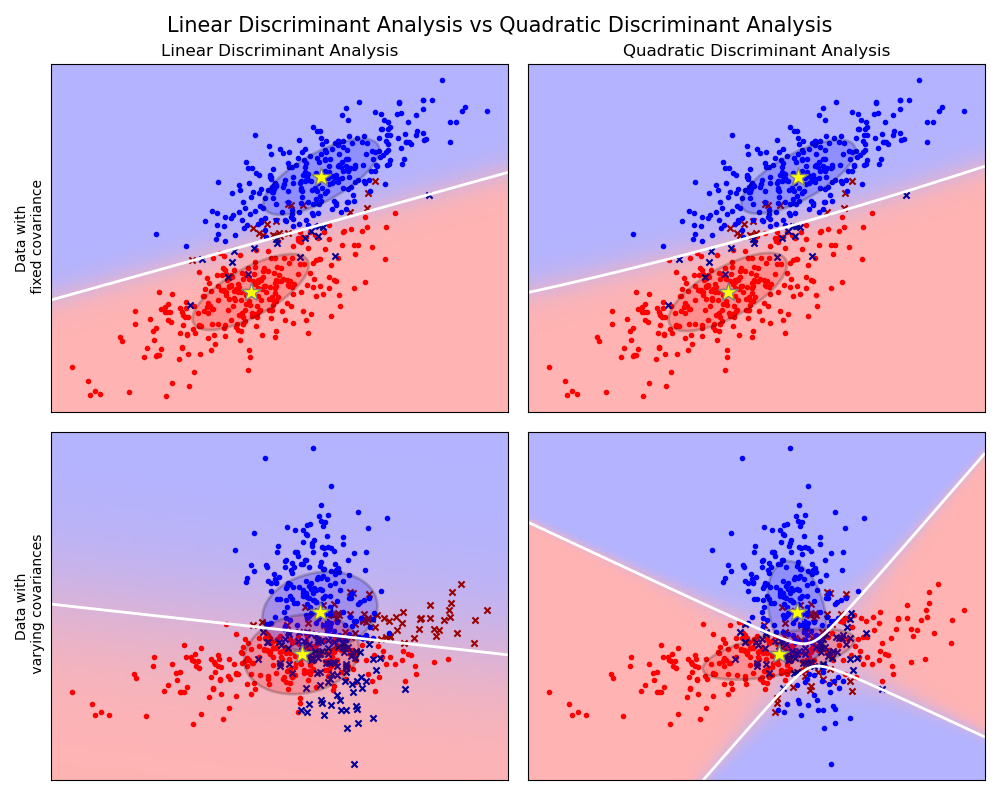## Applications of Gaussian Discriminant Analysis

• Pattern recognition
• Day-to-day prediction (For, e.g., Will it rain today)
• Robots learning classification with the help of Gaussian discriminant analysis.

1. How are LDA and QDA related to GDA?

Ans. Gaussian Discriminant Analysis is an umbrella term for Linear Discriminant and Quadratic Discriminant Analysis. The multivariate Gaussian distribution can model the likelihood probability in both the classifiers.

2.  What is the difference between discriminative and generative learning?

Ans. The generative learning model focuses on classifying the data into various classes and explaining how the data was distributed. On the other hand, the discriminative learning model focus on dividing the data points with the help of a boundary and predicts the labels of other data points.

3. Is Gaussian discriminant analysis unsupervised?

Ans. Gaussian Discriminant analysis belongs to the category of Generative Learning Algorithms, supervised learning. Therefore, GDA is a supervised learning algorithm.

## Key Takeaways

Gaussian Discriminant Analysis classifies the data points in two categories, linear with the help of LDA or non-linear with the use of QDA. This article explained assumptions, classifications, and applications of Gaussian discriminant analysis in detail. If interested in going deeper, Check out our industry-oriented machine learning course curated by our faculty from Stanford University and Industry experts.